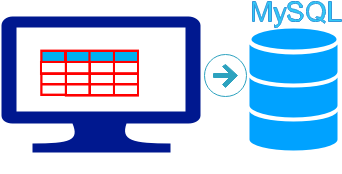# Inserting DataFrame to MySQL table1. Connect to MySQL
2. Create DataFrame
3. Add DataFrame to MySQL table

Inserting DataFrame to MySQL database table by using to_sql() from Excel or CSV sources

## sqlalchemy

We will use SQLAlchemy and its create_engine to manage our database connection from Python to MySQL.
``````from sqlalchemy import create_engine

## Create DataFrame

We will use two columns only to create one DataFrame.
``````import pandas as pd
my_dict={
'class':['Five','Six','Three'],
'No':[5,2,3]
}
df = pd.DataFrame(data=my_dict)``````

## Add Data to MySQL table

We will create one new table student2 and store the DataFrame.
full details with options on to_sql()
Full code is here.
``````import pandas as pd
my_conn=create_engine("mysql+mysqldb://root:test@localhost/my_tutorial")
my_dict={
'class':['Five','Six','Three'],
'No':[5,2,3]
}
df = pd.DataFrame(data=my_dict)
df.to_sql(con=my_conn,name='student2',if_exists='append',index=False)``````

## Reading from table and writing to another table

1. We will read data from one table of MySQL database and using the data we will create one DataFrame.
2.Same DataFrame we will use to create one table using to_sql()

Our sample student table is already available in our Database. First we will collect part of the data ( of class='Five') from this table by using read_sql().
``````import pandas as pd
from sqlalchemy import create_engine
my_conn=create_engine("mysql+mysqldb://root:test@localhost/my_tutorial")
query="SELECT * FROM student WHERE class='Five'"
print(df) # Data taken from table and DataFrame output
df.to_sql(con=my_conn,name='student2',if_exists='append', index=False)``````
The last line in above code will insert the DataFrame to MySQL database in a new table student2.

## Update records with new data

We will read data from student2 table and create a DataFrame. Add 5 marks to each records and replace the new updated data in student2 table.
Watch the option `if_exists='replace'`
``````query="SELECT * FROM student2"
print(df)
df['mark']=df['mark']+5
df.to_sql(con=my_conn,name='student2',if_exists='replace', index=False)``````
To check the updated data we can run this code.
``````query="SELECT * FROM student2"
print(df)``````
Output ( sample)
``````   id         name class  mark gender
0   7  My John Rob  Five    83   male
1   8       Asruid  Five    90   male
2  18        Honny  Five    80   male``````

## Reading Data from csv file and inserting to MySQL table

Place the file in any location and change the path in first line of below code. We used read_csv() to get data and create the DataFrame. After creating DataFrame we are inserting the data into MySQL database table student3. If you are using excel file then use read_excel()
``````student3=pd.read_excel("D:\my_data\student.xlsx")
df=pd.DataFrame(data=student3)
print(df)
df.to_sql(con=my_conn,name='student3', if_exists='append',index=False)``````
If you are using a large file or more number of records then to show a sample records from top we can use head() or to show last records tail().
``````print(df.head())
print(df.tail())``````

Subscribe to our YouTube Channel here

## Subscribe

* indicates required
Subscribe to plus2netplus2net.com